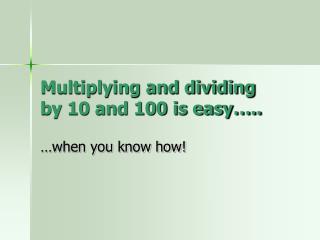DownloadDownload PresentationMultiplying and dividing by 10 and 100 is easy…..

# Multiplying and dividing by 10 and 100 is easy…..

Télécharger la présentation## Multiplying and dividing by 10 and 100 is easy…..

- - - - - - - - - - - - - - - - - - - - - - - - - - - E N D - - - - - - - - - - - - - - - - - - - - - - - - - - -
##### Presentation Transcript

1. Multiplying and dividing by 10 and 100 is easy….. …when you know how!

2. Make sure you keep an eye on the place – value markers…they’re REALLY important. Th H T U . t h 3 2 . 1 0 Multiply it by 10, so every digit becomes 10 times BIGGER. EVERY digit moves one place to the LEFT. Th H T U . t h 3 2 . 1 0 3 2 1 . 0

3. Lets look at that again… • We’ll multiply 56.03 by 10. • Remember what happens to EACH digit. • Th H T U . t h • 5 6 . 0 3 X10 • 5 6 0 . 3 • Check that the order of the digits is the same as when you started.

4. So what happens when you multiply by 100…let’s see • TH H T U . t h • 4 6 . 0 2 X 100 4 6 0 2 . • See! Each digit moves across two places to the left…and of course • 100 has 2 zeros, so that’s a good reminder. The number becomes 100 times bigger

5. Now if you make numbers bigger by moving them to the left…what do you think happens when you divide numbers by 10? • Yes… you move each digit one place to the right, to make it 10 times smaller! • Like this… • TH H T U . t h • 3 5 0 . 0 • 3 5 . 0 0 • REALLY IMPORTANT!!!!!!!!!! See how the decimal point stays inn the same place.

6. So now you can guess what happens when you divide by 100. • That’s right, every digit moves two places to the right to make the number 100 times smaller. • Lets divide 12.6 into 100 bits…wow how tiny would each bit be? • TH H T U . t h th t.th • 1 2 . 6 0 divided by 100 • 0 . 1 2 6 0 • So 0.1260 is 100 times smaller than 12.6